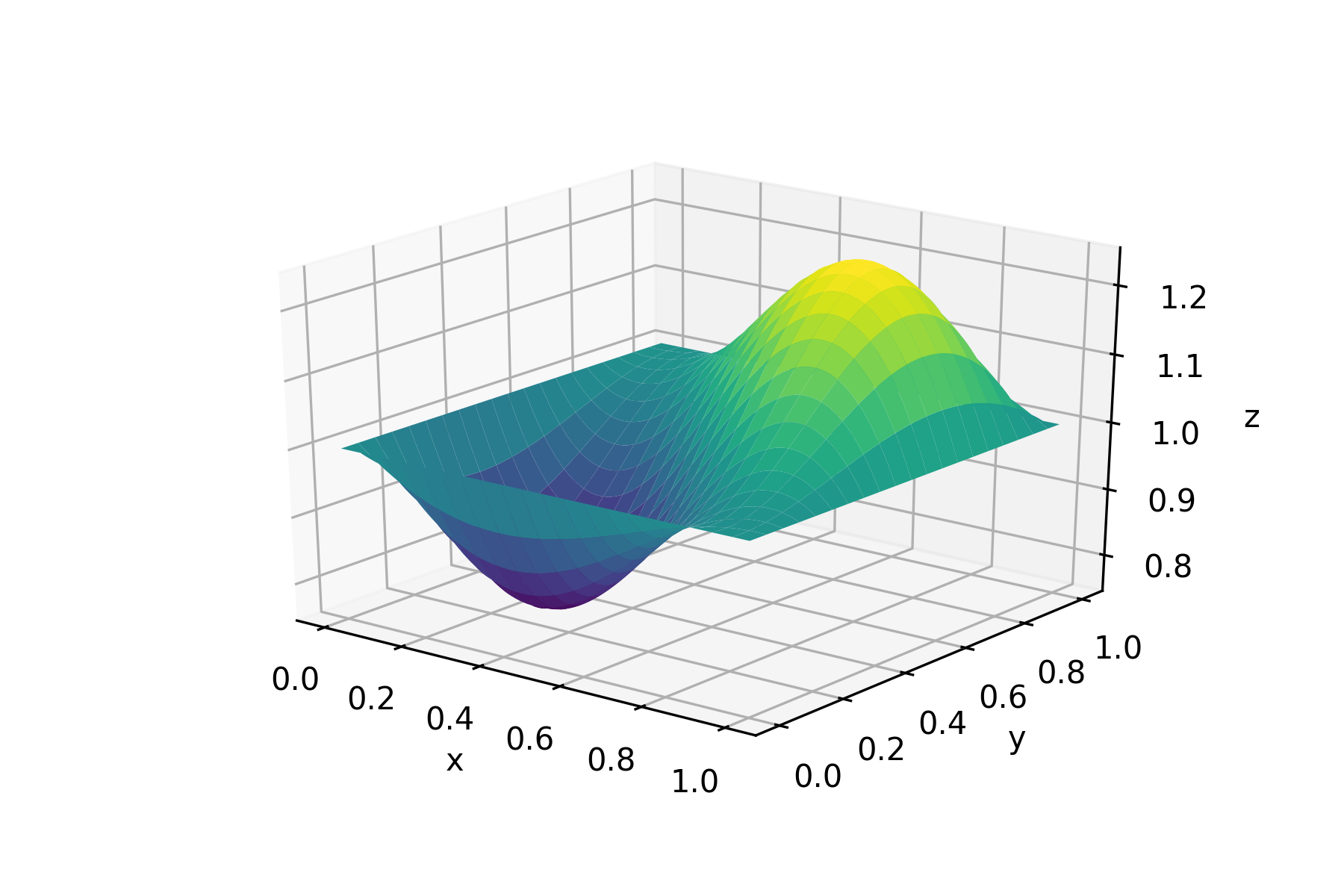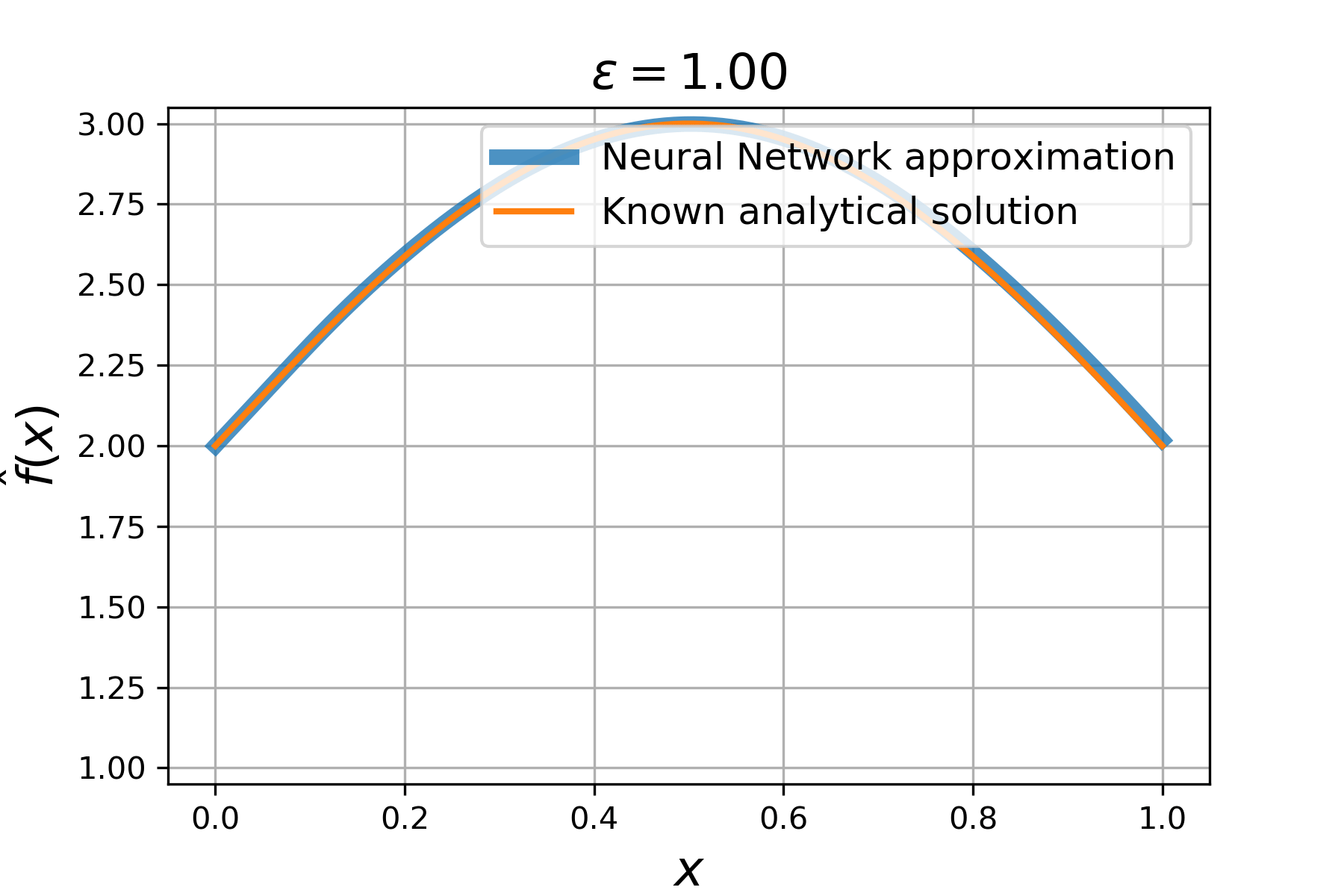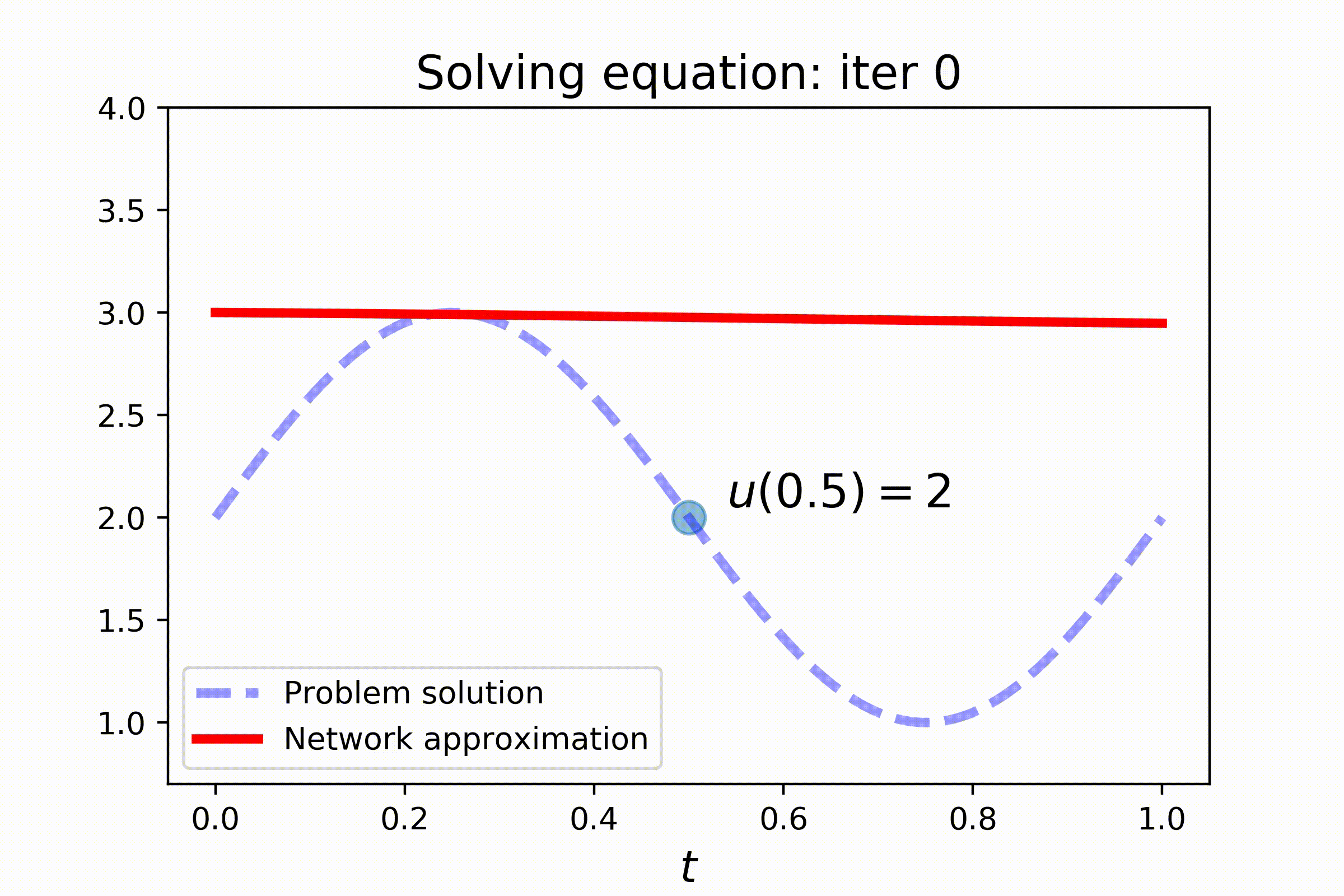pydens
pypi i pydens

pypi i pydens

# PyDEns

PyDEns is a framework for solving Ordinary and Partial Differential Equations (ODEs & PDEs) using neural networks. With PyDEns one can solve

This page outlines main capabilities of PyDEns. To get an in-depth understanding we suggest you to also read the tutorial.

## Getting started with PyDEns: solving common PDEs

Let's solve poisson equationusing simple feed-forward neural network. Let's start by importing `Solver`-class along with other needed libraries:

``````from pydens import Solver, NumpySampler
import numpy as np
import torch

``````

You can now set up a PyDEns-model for solving the task at hand. For this you need to supply the equation into a `Solver`-instance. Note the use of differentiation token `D`:

``````# Define the equation as a callable.
def pde(f, x, y):
return D(D(f, x), x) + D(D(f, y), y) - 5 * torch.sin(np.pi * (x + y))

# Supply the equation, initial condition, the number of variables (`ndims`)
# and the configration of neural network in Solver-instance.
solver = Solver(equation=pde, ndims=2, boundary_condition=1,
layout='fa fa fa f', activation='Tanh', units=[10, 12, 15, 1])

``````

Note that we defined the architecture of the neural network by supplying `layout`, `activation` and `units` parameters. Here `layout` configures the sequence of layers: `fa fa fa f` stands for `f`ully connected architecture with four layers and three `a`ctivations. In its turn, `units` and `activation` cotrol the number of units in dense layers and activation-function. When defining neural network this way use `ConvBlock` from `BatchFlow`.

It's time to run the optimization procedure

``````solver.fit(batch_size=100, niters=1500)
``````

in a fraction of second we've got a mesh-free approximation of the solution on [0, 1]X[0, 1]-square:## Going deeper into PyDEns-capabilities

PyDEns allows to do much more than just solve common PDEs: it also deals with (i) parametric families of PDEs and (ii) PDEs with trainable coefficients.

### Solving parametric families of PDEs

Consider a family of ordinary differential equationsClearly, the solution is a sin wave with a phase parametrized by ϵ:Solving this problem is just as easy as solving common PDEs. You only need to introduce parameter `e` in the equation and supply the number of parameters (`nparams`) into a `Solver`-instance:

``````def odeparam(f, x, e):
return D(f, x) - e * np.pi * torch.cos(e * np.pi * x)

# One for argument, one for parameter
s = NumpySampler('uniform') & NumpySampler('uniform', low=1, high=5)

solver = Solver(equation=odeparam, ndims=1, nparams=1, initial_condition=1)
solver.fit(batch_size=1000, sampler=s, niters=5000, lr=0.01)
# solving the whole family takes no more than a couple of seconds!
``````

Check out the result:### Solving PDEs with trainable coefficients

With PyDEns things can get even more interesting! Assume that the initial state of the system is unknown and yet to be determined:Of course, without additional information, the problem is undefined. To make things better, let's fix the state of the system at some other point:Setting this problem requires a slightly more complex configuring. Note the use of `V`-token, that stands for trainable variable, in the initial condition of the problem. Also pay attention to the additional constraint supplied into the `Solver` instance. This constraint binds the final solution to zero at `t=0.5`:

``````def odevar(u, t):
return D(u, t) - 2 * np.pi * torch.cos(2 * np.pi * t)
def initial(*args):
return V('init', data=torch.Tensor([3.0]))

solver = Solver(odevar, ndims=1, initial_condition=initial,
constraints=lambda u, t: u(torch.tensor([0.5])))
``````

When tackling this problem, `pydens` will not only solve the equation, but also adjust the variable (initial condition) to satisfy the additional constraint. Hence, model-fitting comes in two parts now: (i) solving the equation and (ii) adjusting initial condition to satisfy the additional constraint. Inbetween the steps we need to freeze layers of the network to adjust only the adjustable variable:

``````solver.fit(batch_size=150, niters=100, lr=0.05)
solver.model.freeze_layers(['fc1', 'fc2', 'fc3'], ['log_scale'])
solver.fit(batch_size=150, niters=100, lr=0.05)
``````

Check out the results:## Installation

First of all, you have to manually install pytorch, as you might need a certain version or a specific build for CPU / GPU.

### Stable python package

With modern pipenv

``````pipenv install pydens
``````

With old-fashioned pip

``````pip3 install pydens
``````

### Development version

``````pipenv install git+https://github.com/analysiscenter/pydens.git
``````
``````pip3 install git+https://github.com/analysiscenter/pydens.git
``````

### Installation as a project repository:

Do not forget to use the flag `--recursive` to make sure that `BatchFlow` submodule is also cloned.

``````git clone --recursive https://github.com/analysiscenter/pydens.git
``````

In this case you need to manually install the dependencies.

## Citing PyDEns

``````Roman Khudorozhkov, Sergey Tsimfer, Alexander Koryagin. PyDEns framework for solving differential equations with deep learning. 2019.
``````
``````@misc{pydens_2019,
author       = {Khudorozhkov R. and Tsimfer S. and Koryagin. A.},
title        = {PyDEns framework for solving differential equations with deep learning},
year         = 2019
}
``````

165

2yrs ago

1

5

8

### OPEN PRs

0
VersionTagPublished
1.0.2
7mos ago
1.0.0
7mos ago
0.1.2
3yrs ago
0.1.1
3yrs ago